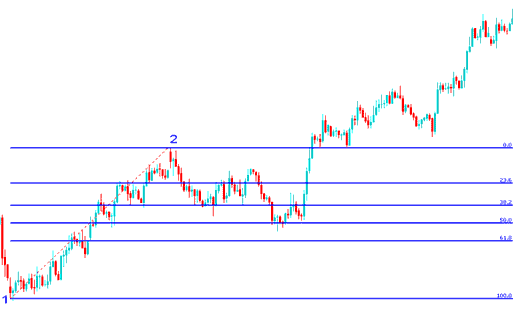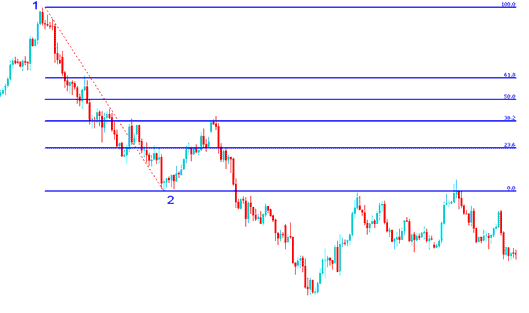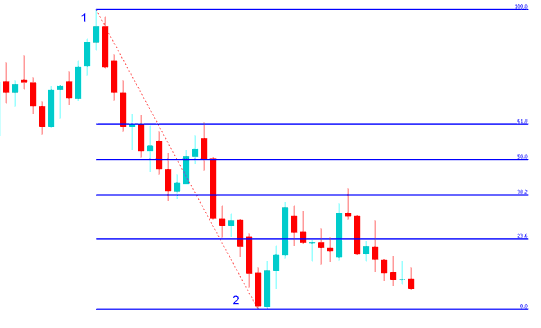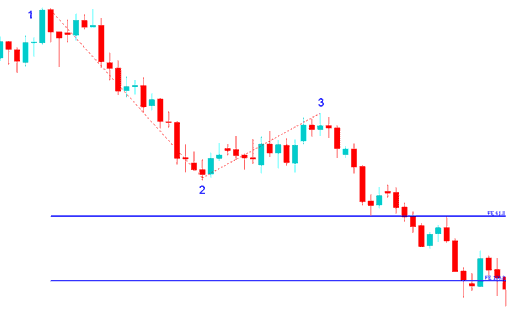# Fibonacci Drawing on Gold Trading Charts Exercises

These exercises will give more examples on trading and drawing Fibonacci retracement levels and Fibonacci expansion levels. The first exercise is to figure out the direction of the Gold price trend and then determine if the Fibonacci tool used is Fibonacci retracement or Fibonacci expansion and then figure how it is drawn then practice drawing them on a Gold trading chart using a practice demo account.

Figure out the direction of the Gold price trend

Determine if the Fibonacci is retracement or expansion

Figure how it is drawn

Practice drawing retracement or expansion on a XAUUSD Gold price chart.

To find XAUUSD gold trading charts just download MetaTrader 4 Gold trading platform from any one of the many online brokers and select the financial instrument - XAUUSD.

After you have downloaded the MetaTrader 4 platform, Go to the menu "insert" at the top left corner, on the drop down menu, click Fibonacci and select either the option of retracement or expansion. Then draw it as explained in the previous lessons of Fibonacci Expansion and Fibonacci Retracement. Then do the following exercises:

Fibonacci Retracement Levels and Fibonacci Expansion Levels Exercise

## Exercises 1:## Exercises 2:## Exercises 3:## Exercises 4:Hint: point 1 to point 2 follows the trend direction

Hint: Retracement has points 1 and 2

Hint: Expansion has point 1, 2 and 3